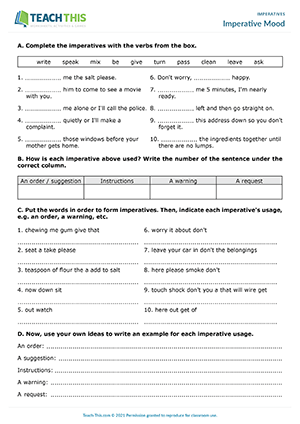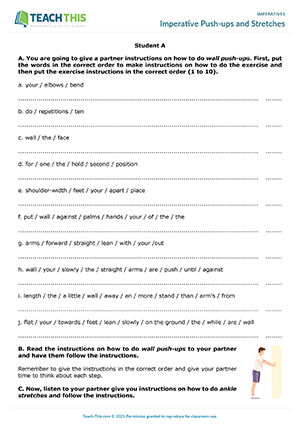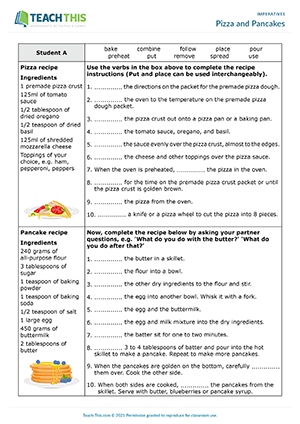# Imperatives Worksheets and ESL Activities

## Classroom Commands

### ESL Classroom Imperatives Worksheet - Reading, Matching and Writing Activity - Elementary (A1-A2) - 30 minutes

This printable imperatives worksheet helps students to learn and practice classroom commands and language. Give each student a copy of the two-page worksheet. After reading through how the imperative is used and formed, students match classroom commands to pictures and then write them in the boxes underneath the pictures. Next, students connect sentence halves together to form imperatives. After that, students put words in order to make imperatives related to classroom commands. In the last exercise, students think about what rules they have in their classroom and write them down in the imperative form. When the students have finished, review their answers together as a class and give feedback.Interactive Version - In this classroom imperatives interactive PDF worksheet, students complete a variety of exercises to learn imperatives, classroom commands and language.

## Imperative Mood

### ESL Imperative Mood Worksheet - Reading and Writing Activity - Pre-intermediate (A2) - 25 minutes

This printable imperatives worksheet helps students learn how the imperative mood is used for orders, instructions, suggestions, warnings and requests. Give each student a copy of the worksheet. Students begin by completing imperatives with verbs from a box. Students then sort the sentences, according to how each imperative is used. Next, students put words in the correct order to form imperatives. The students then indicate each imperative's usage, e.g. an order, a warning, etc. In the last exercise, students use their own ideas to write an example for each imperative usage. When the students have finished, they read their examples to the class.Interactive Version - This interactive imperative exercises PDF helps students learn how the imperative mood is used for orders, instructions, suggestions, warnings and requests.

## Imperative Push-ups and Stretches

### ESL Imperatives Activity - Reading, Writing, Listening and Speaking - Pre-intermediate (A2) - 25 minutes

In this entertaining imperatives activity, students put instructions in order and then take it in turns to give and follow instructions with a partner. Divide the students into two groups (A and B) and give each student a corresponding worksheet. The students' task is to give a partner instructions on how to do something, but first they must put the instructions in order. Students look at their worksheet and put the words in each sentence in order. Then, they write numbers (1 to 10) on the lines next to the sentences to put the instructions in the correct order. When the students have finished, check the answers with each group and the students identify the imperative verbs. Students then pair up with someone from the other group. Next, Student A reads their step-by-step instructions to Student B who listens and follows what is said. Afterwards, the two students swap roles so that each student has a turn reading and following a set of instructions.## Imperatives

### ESL Imperatives Worksheet - Reading and Writing Activity - Pre-intermediate (A2) - 30 minutes

Here is a free imperatives worksheet for pre-intermediate students. Give each student a copy of the two-page worksheet. Students begin by writing 'I' (for imperative) next to sentences that contain imperatives. Check the answers and then have the students rewrite the unmarked sentences from Exercise A as imperatives. Students then move on to do a gap fill exercise where they complete imperatives with words from a box and then underline the imperatives in each sentence. In the last exercise, students circle the correct form of the verbs in bold and then underline the imperatives.Interactive Version - In this free imperatives interactive worksheet, students complete a range of exercises to learn and practice imperatives.

## Let's Practice Imperatives

### ESL Imperatives Worksheet - Reading, Matching and Writing Activity - Pre-intermediate (A2) - 30 minutes

This printable imperatives worksheet helps students to learn and practice the imperative, including the use of let's to include yourself in the imperative. Give each student a copy of the two-page worksheet. Students begin by matching sentences that go together. One or both sentences in each pair uses the imperative. Students then underline the imperative verbs in the sentences. Next, students choose words and phrases from a box to complete imperative sentences. Students then move on to read statements and then write what each person says using imperatives. In the last exercise, students complete each imperative with their own ideas. Then, they write another sentence to give more information about it. Afterwards, review the students' answers as a class.Interactive Version - In this imperatives with Let's interactive worksheet, students do a variety of exercises to help them learn and practice imperatives and the use of imperatives with let's.

## Pizza and Pancakes

### ESL Imperatives Activity - Reading, Writing, Listening and Speaking - Intermediate (B1) - 25 minutes

This engaging imperatives activity provides students with practice using the imperative while completing instructions on pizza and pancake recipes. Divide the students into two groups (A and B) and give each student a corresponding worksheet. The students look at the top recipe on their worksheet and use the verbs in the box to complete the recipe instructions. When the students have finished, check the answers with each group. Next, students pair up with someone from the other group. Students then take it in turns to complete the second recipe on their worksheet by asking their partner questions, e.g. 'What do you do with the butter?' The students should ask at least one question for each missing verb. If students have problems coming up with a question, they can ask questions like 'What do you do next?' When both students have completed the second recipe, they compare the two recipes and discuss similarities and differences between them.0
0
0
s2sdefault